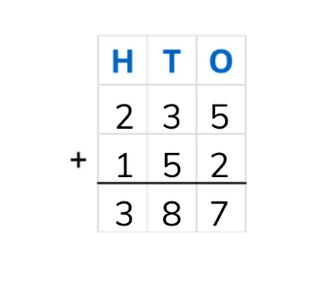Addition to 1,000 using standard algorithm

# Addition to 1,000 using standard algorithm

Students learn to add numbers to 1,000 using the standard algorithm.8,000 schools use Gynzy92,000 teachers use Gynzy1,600,000 students use Gynzy

## General

Students are able to add numbers to 1,000 using the standard algorithm.

4.NBT.B.4

## Relevance

Using the standard algorithm allows you to add numbers that are difficult to add in one step in your head. You can use the standard algorithm to add larger numbers together.

## Introduction

Ask students to create the largest possible number using the digits given on the interactive whiteboard. Ask students to name the place value of the numbers in their locations (thousands, hundreds, tens, ones).

## Development

Check that students are able to add numbers to 1,000 using the standard algorithm by asking the following questions:
- Why is it useful to be able to use the standard algorithm?
- What do the letters H, T, and O stand for?
- Where do you start adding when using the standard algorithm?
- Solve the following problems using the standard algorithm: 128 + 431 = ?, and 556 + 243 = ?

## Guided practice

Students are given addition problems in which they must solve their problems in the place value chart. They must also write the addition problems in the place value chart and then solve for the total.

## Closing

Ask students to solve the math problems shown in the cupcakes using the standard algorithm, writing the numbers one below the other. Then drag the correct cupcake (answer) to the problem. Discuss why it is useful to be able to use the standard algorithm and how it works. Remind students that the standard algorithm works from right to left. First add the ones, then the tens, and then the hundreds. You can use this to quickly and easily solve addition problems with larger numbers.

## Teaching tips

To support students who have difficulty with the standard algorithm, start by working on their knowledge of the place value chart (HTO) and how to correctly write a number into the place value chart. You can choose to write the addition problems of the ones, tens, hundreds separately and then to write those totals together to make it extra clear which numbers are being added together.

### The online teaching platform for interactive whiteboards and displays in schools

• Save time building lessons

• Manage the classroom more efficiently

• Increase student engagement